石油地球物理勘探2021, Vol. 56Issue (2): 313-322  DOI: 10.13810/j.cnki.issn.1000-7210.2021.02.0130文章快速检索 高级检索

### 引用本文LUO Tengteng, XU Jixiang, SUN Xiping. Diffraction wave separation and imaging based on high-resolution Radon transform on an iterative model shrinking approach. Oil Geophysical Prospecting, 2021, 56(2): 313-322. DOI: 10.13810/j.cnki.issn.1000-7210.2021.02.013.### 作者简介罗腾腾, 北京市海淀区学院路20号中国石油勘探开发研究院, 100083。Email: ttluomail@163.com

### 文章历史

Diffraction wave separation and imaging based on high-resolution Radon transform on an iterative model shrinking approach
LUO Tengteng, XU Jixiang , SUN Xiping
Research Institute of Petroleum Exploration and Development, PetroChina, Beijing 100083, China
Abstract: In order to make full use of the diffractions in seismic record to describe small underground structures (faults, fractures, holes, etc.), it is necessary to separate weak diffractions from seismic full-wave field and image diffractions separately. In dip-angle common imaging point gathers (CIGs), a separating and imaging method of diffractions based on high-resolution Radon transform on an iterative model shrinking approach is proposed. This method considers the morphological difference in diffracted and reflected events. It uses an iterative shrinkage threshold algorithm (ISTA) to obtain the sparsity of Radon model through model shrinkage steps in time domain and solve the problem that the separated effect of diffraction wave field caused by poor focusing of reflection and diffraction energy group is not ideal in conventional Radon transform. On the basis of programming algorithm, the method can effectively separate diffracted waves and has strong anti-noise ability on model data. On real data, the computing efficiency of the method is about twice than that of the conventional IRLS at more than 20 iterations, proving it applicable for real seismic data.
Keywords: Radon transform    iterative model shrin-kage    high resolution    dip-angle CIGs    diffraction separation and imaging
0 引言

(2) 在叠加过程中进行绕射波分离与成像。Kanasewich等直接对地震信号沿着绕射波走时曲线进行叠加，获得了共炮检距道集和共断层点道集上的绕射波时间剖面。Berkovich等、Dell等分别利用绕射波多聚焦和共反射面的绕射走时计算方法，将选取的绕射波同相轴进行最优叠加，得到包含绕射波的叠加剖面。Asgedom等利用修正的共反射面(Common Reflection Surface，CRS)技术讨论了相似度和多信号分类两种方法在分离反射波与绕射波时的优势。

(3) 在偏移过程中进行绕射波分离与成像。Landa等推导了深度偏移倾角域共成像点道集(Common Imaging Gathers，CIGs)中反射波和绕射波的形态，并分析了偏移速度对两者的影响。Zhu等在小波束偏移过程中，利用角度域局部成像矩阵中反射与绕射能量的分布差异，即反射能量沿着倾角方向呈线性分布而绕射能量弥散分布在整个矩阵中，分离出绕射能量并对其单独成像。Klokov等将相似度扫描反射顶点去除与混合Radon变换方法相结合，在倾角域CIGs上进行绕射波场分离。Decker等在偏移倾角道集中，对每个固定倾角的成像剖面应用PWD解构滤波技术，获得单独的绕射波成像剖面。Zhang等提出在单炮的反射角域CIGs中通过切除菲涅耳带提取绕射波场。黄建平等和李振春等将逆时偏移与最小二乘偏移的优势相结合，分别发展了适用于复杂构造的保幅成像处理方法。刘梦丽等提出一种满足能量守恒的逆散射成像条件，并将其与最小二乘逆时偏移(Reverse Time Migration，RTM)相结合，实现了角度滤波成像。汪天池等在倾角域中利用逆时偏移提取倾角道集，然后采用中值滤波方法实现反射波与绕射波的分离。

1 方法原理 1.1 倾角域共成像点道集波场特征

 $z(x)=z_{1}+x \tan \alpha_{0}$ (1)图 1 零炮检距反射示意图

 $w(y)=\frac{2\left(z_{1} \cos \alpha_{0}+y \sin \alpha_{0}\right)}{v}$ (2)

 $x_{\mathrm{m}}=y-\frac{v_{\mathrm{m}} w}{2} \sin \alpha$ (3)
 $\tau=w \cos \alpha$ (4)

 $\tau\left(x_{\mathrm{m}}, \alpha\right)=\frac{2 \cos \alpha}{v} \times \frac{x_{\mathrm{m}} \sin \alpha_{0}+z_{1} \cos \alpha_{0}}{1-\gamma \sin \alpha_{0} \sin \alpha}$ (5)

 $x_{\mathrm{m}} \sin \alpha_{0}+z_{1} \cos \alpha_{0}=z_{0} \cos \alpha_{0}$ (6)

 $\tau(\alpha)=\tau_{0} \frac{\cos \alpha_{0} \cos \alpha}{1-\gamma \sin \alpha_{0} \sin \alpha}$ (7)

 $\frac{\partial \tau(\alpha)}{\partial \alpha}=\tau_{0} \cos \alpha_{0} \frac{\gamma \sin \alpha_{0}-\sin \alpha}{\left(1-\gamma \sin \alpha_{0} \sin \alpha\right)^{2}}$ (8)

 $\tau(\alpha)=\tau_{0} \cos \alpha \frac{\xi \gamma \sin \alpha+\sqrt{\xi^{2}+1-\gamma^{2} \sin ^{2} \alpha}}{1-\gamma^{2} \sin ^{2} \alpha}$ (9)

 $\tau(\alpha)=\tau_{0}$ (10)

 $z(\alpha)=z_{0} \gamma \frac{\cos \alpha_{0} \cos \alpha}{1-\gamma \sin \alpha_{0} \sin \alpha}$ (11)

 $z(\alpha)=\gamma \cos \alpha \frac{\rho \gamma \sin \alpha+\sqrt{\rho^{2}+z_{0}^{2}\left(1-\gamma^{2} \sin ^{2} \alpha\right)}}{1-\gamma^{2} \sin ^{2} \alpha}$ (12)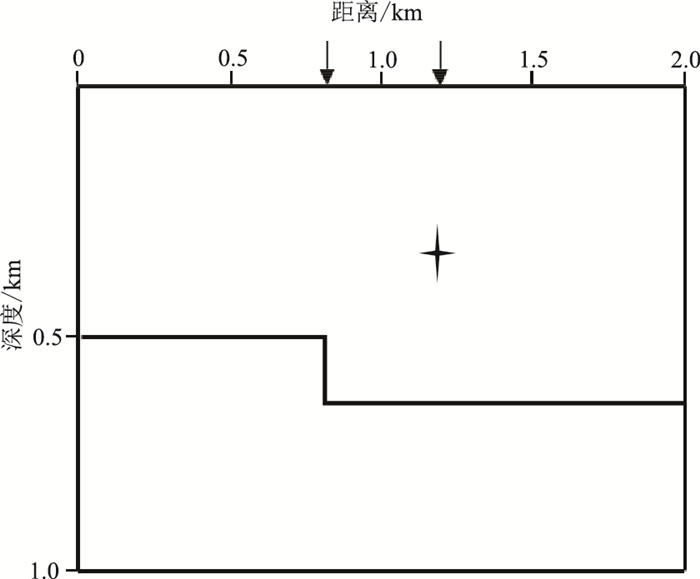图 2 常速介质模型 垂直断层和绕射体分别位于0.8km和1.2km的横向位置；“十”字符号示意绕射目标体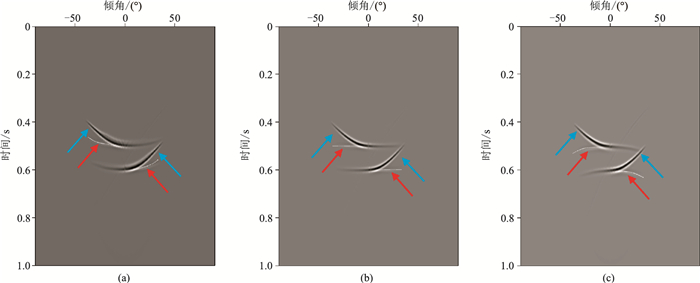图 3 不同偏移速度误差时断层处的倾角域CIGs (a)偏移速度为介质速度的90%；(b)偏移速度等于介质速度；(c)偏移速度为介质速度的110%蓝色和红色箭头分别指示反射波和绕射波同相轴，图 4同图 4 不同偏移速度误差时绕射体处的倾角域CIGs (a)偏移速度为介质速度的90%；(b)偏移速度等于介质速度；(c)偏移速度为介质速度的110%

 $d(t, u)=\int_{-\infty}^{+\infty} m\left(\tau=t-q u^{2}, q\right) \mathrm{d} q$ (13)

 $\boldsymbol{D}\left(\omega, u_{k}\right)=\sum\limits_{r=0}^{n-1} \boldsymbol{M}\left(\omega, q_{r}\right) \mathrm{e}^{-\mathrm{i} \omega q_{r} u_{k}{}^{2}}$ (14)

 $\mathit{\boldsymbol{d}} = \mathit{\boldsymbol{Lm}}$ (15)

 $J=\|\boldsymbol{d}-\boldsymbol{L} \boldsymbol{m}\|_{s}^{s}+\beta\|\boldsymbol{m}\|_{p}^{p}$ (16)

 $J=\|\boldsymbol{d}-\boldsymbol{L m}\|_{2}^{2}+\beta\|\boldsymbol{m}\|_{2}^{2}$ (17)

 $\boldsymbol{m}=\left(\boldsymbol{L}^{\mathrm{T}} \boldsymbol{L}+\boldsymbol{\beta} \boldsymbol{I}\right)^{-1} \boldsymbol{L}^{\mathrm{T}} \boldsymbol{d}$ (18)

 $J=\|\boldsymbol{d}-\boldsymbol{L} \boldsymbol{m}\|_{2}^{2}+\beta\|\boldsymbol{m}\|_{1}^{1}$ (19)

 $\begin{array}{l} {\mathit{\boldsymbol{m}}_k} = {T_\sigma }\left\{ {{\mathit{\boldsymbol{m}}_{k - 1}} + 2{t_0}{{\rm{F}}^{ - 1}}\left\{ {{{({\mathit{\boldsymbol{L}}^{\rm{T}}}\mathit{\boldsymbol{L}})}^{ - 1}}{\mathit{\boldsymbol{L}}^{\rm{T}}}\left[ {{\rm{F}}(\mathit{\boldsymbol{d}}) - } \right.} \right.} \right.\\ {\kern 1pt} {\kern 1pt} {\kern 1pt} {\kern 1pt} {\kern 1pt} {\kern 1pt} {\kern 1pt} {\kern 1pt} {\kern 1pt} {\kern 1pt} {\kern 1pt} {\kern 1pt} {\kern 1pt} {\kern 1pt} {\kern 1pt} {\kern 1pt} \left. {\left. {\mathit{\boldsymbol{L}}{\rm{F}}\left( {{\mathit{\boldsymbol{m}}_{k - 1}}} \right)} \right]} \right\} \end{array}$ (20)

 $T_{\sigma}\{\boldsymbol{m}, k\}_{i j}=\left(\left|m_{i j}\right|-\alpha \frac{K-k}{K} \tilde{m}_{i j}\right)_{+} \operatorname{sgn}\left(m_{i j}\right)$ (21)

 $(X)_{+}=\left\{\begin{array}{ll} X & X \geqslant 0 \\ 0 & X<0 \end{array}\right.$ (22)

(1) 给定阈值σ、最大迭代次数K和二维中值滤波器的大小以及初始迭代步长t1，将当前迭代次数k设置为0。

(3) 在迭代变量k=1，2，...，K时，执行下述迭代过程：

① 将上次的模型值mk-1傅里叶变换到频率域得到F(mk-1)；

③ 计算频率域中数据拟合误差F(d)-LF(mk-1)；

④ 计算算子的伪逆矩阵(LTL)-1LT与数据拟合误差的乘积，即(LTL)-1LT[F(d)-LF(mk-1)]；

⑤ 利用傅里叶反变换将频率域数据变换到时间域，即F-1{(LTL)-1LT[F(d)-LF(mk-1)]}；

⑥ 利用带有收缩运算的方程Tσ计算模型更新值mk

(4) 循环增加迭代次数k，检查是否达到了最大迭代次数，如果“否”，则转到第(3)步。

2 模型试算 2.1 有效性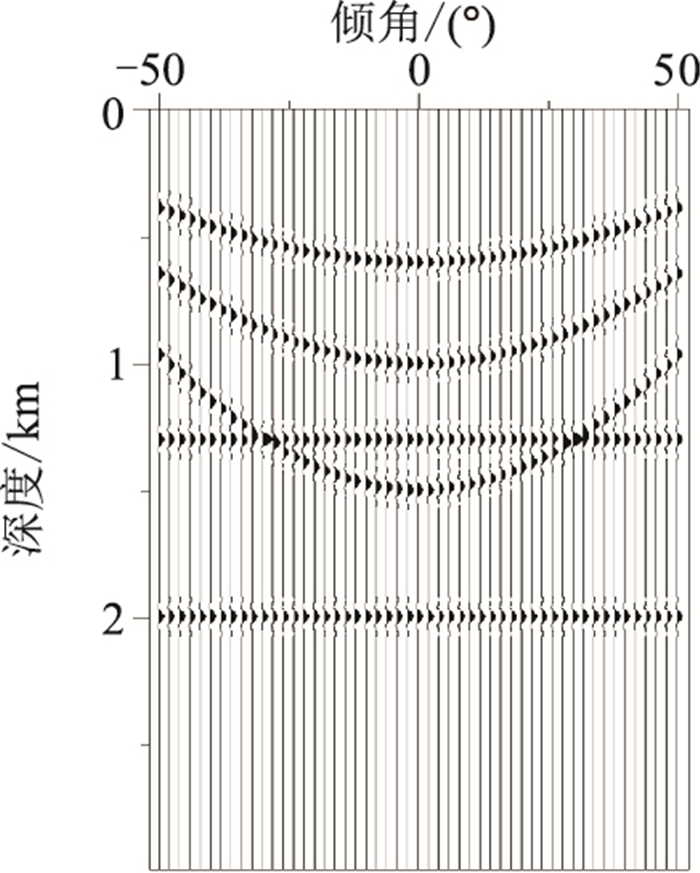图 5 模拟数据的CIG记录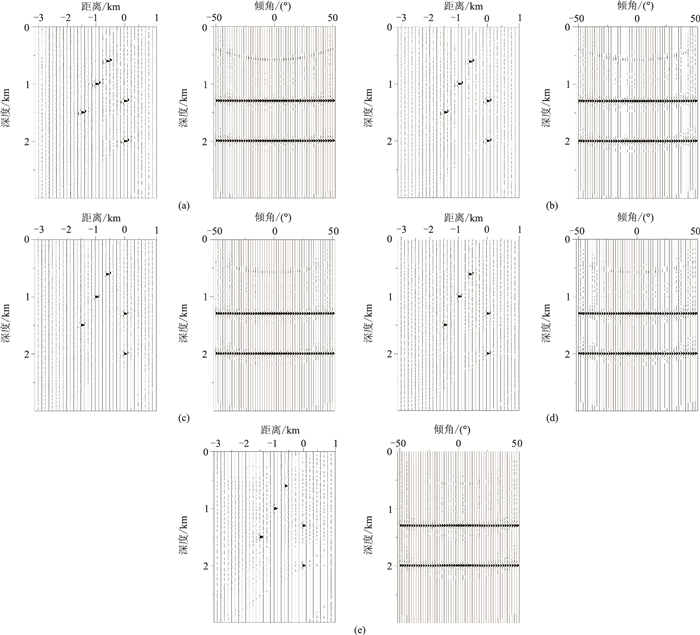图 6 不同迭代次数下迭代收缩高分辨率Radon变换结果 (a)1；(b)5；(c)10；(d)15；(e)20。左为Radon域全波场，右为时空域绕射波波场

2.2 抗噪能力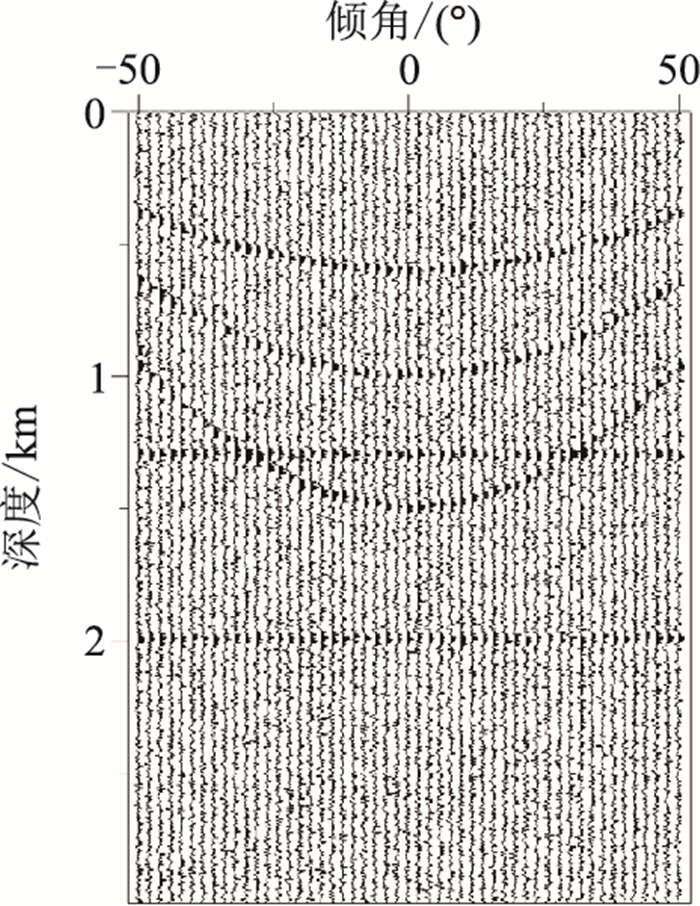图 7 包含高斯白噪的模拟CIG记录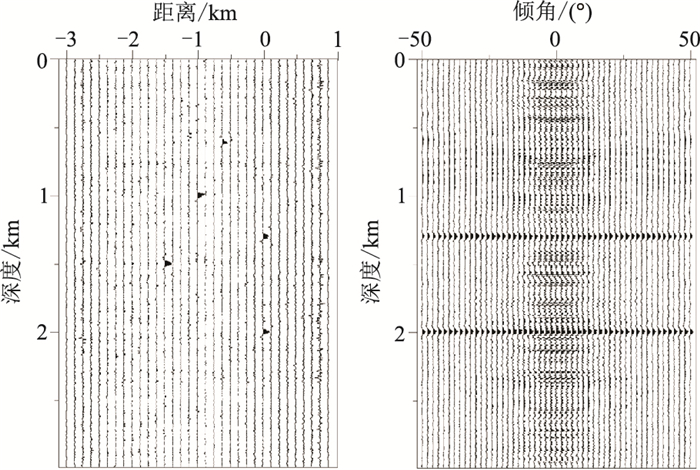图 8 常规最小二乘Radon变换结果 左为Radon域全波场，右为时空域绕射波波场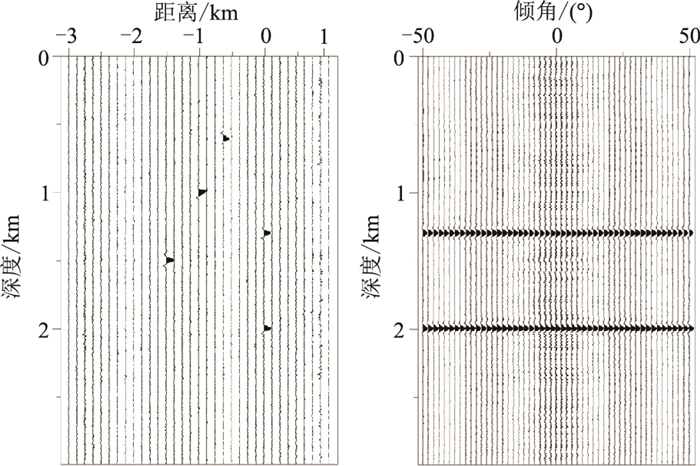图 9 迭代收缩高分辨率Radon变换结果 左为Radon域全波场，右为时空域绕射波波场
2.3 计算效率表 1 两种Radon变换方法运算时间 s
3 实际资料应用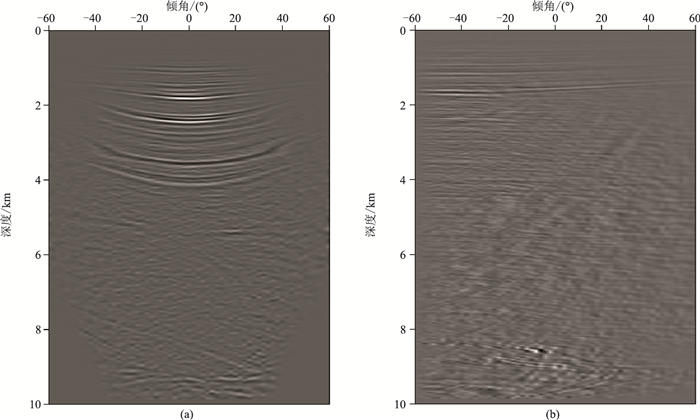图 10 基于迭代高收缩分辨率Radon变换的CIGs波场分离 (a)实际地震资料的全波场CIGs；(b)波场分离后的绕射波CIGs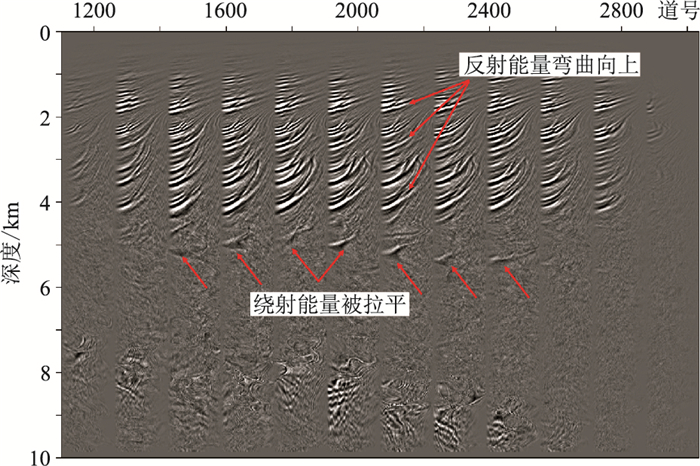图 11 Inline1320测线倾角域CIGs(0~60°)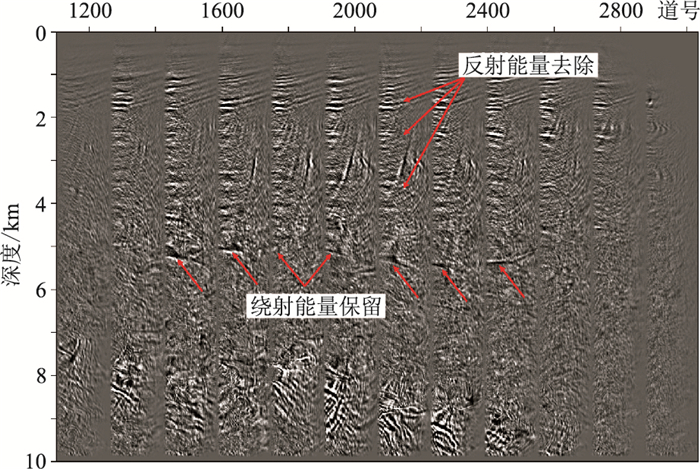图 12 Inline1320测线Radon变换后的倾角域CIGs(0~60°)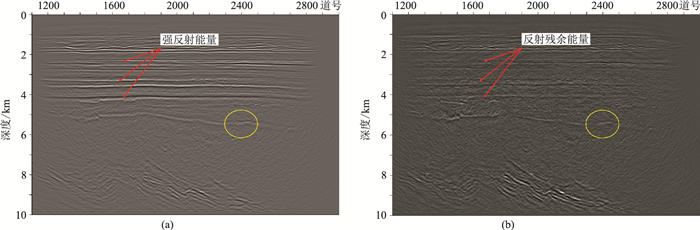图 13 中国西部M探区实际资料(Inline1320测线)处理结果 (a)波场分离前PSDM剖面；(b)波场分离后绕射波成像剖面
4 结论

(1) 在倾角域CIGs中，反射波同相轴总是呈现开口向上的“笑脸”状曲线，绕射同相轴则表现为拟线性，根据两者曲线形态的差异可以实现波场分离；